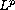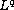Electron. J. Diff. Eqns., Vol. 2005(2005), No. 74, pp. 1-17.

###-estimates for damped wave equations with odd initial data Takashi Narazaki

Abstract:
We study the Cauchy problem for the damped wave equation. In a previous paper  the author has shown the-estimates between the solutions of the damped wave equation and the solutions of the corresponding heat equation. In this paper, we show new-estimates for the damped wave equation with odd initial data.

Submitted July 5, 2003. Published July 5, 2005.
Math Subject Classifications: 35B40, 35L05.
Key Words: Damped wave equation;-estimate; odd initial data

Show me the PDF file (306K), TEX file, and other files for this article.Takashi Narazaki Department of Mathematical Sciences Tokai University Hiratsuka 259-1292, Japan email: narazaki@ss.u-tokai.ac.jp